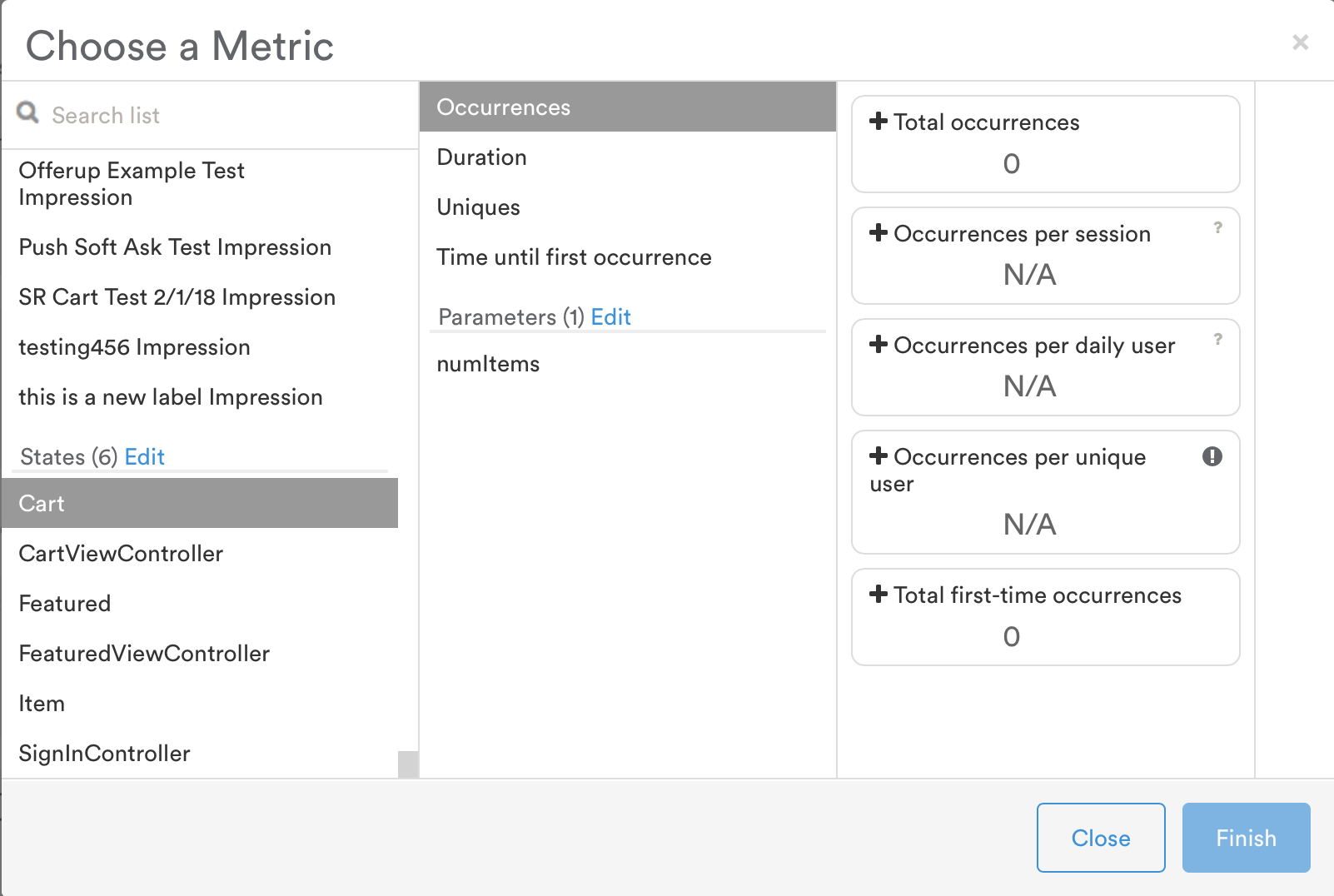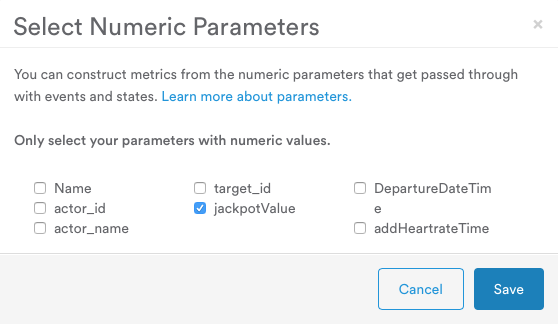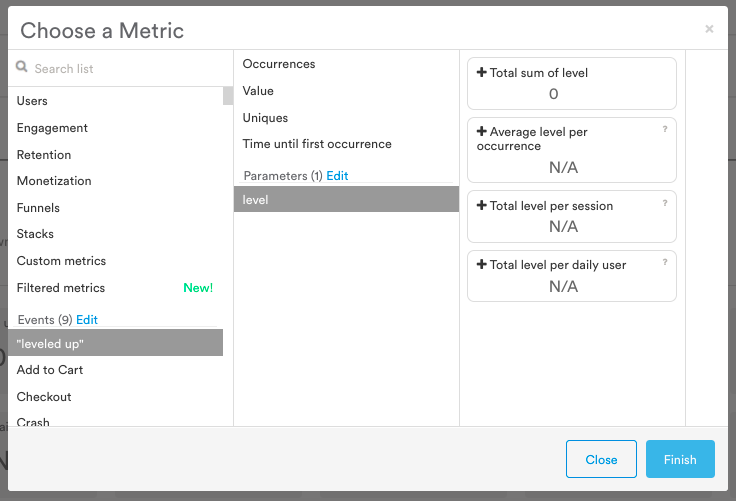# State metrics

Metrics tracked for states and their parameters## Available metrics

Your custom states tracked in Leanplum create the following metrics.

### Occurrences

Metric

Definition

Total occurrences

The total number of times this state was reached.

Occurrences per session

The average number of times this state is reached per session.
(Total # of Occurrences) / (Total Sessions)

Occurrences per daily user

The average number of times a user reaches this state.
(Total # of Occurrences) / (Total Daily Users)

Total first-time occurrences

The total number of times this state was reached for the first time.

### Duration

Metric

Definition

Total time in state

The total time all users spent in this state.

Average duration

The average duration of time spent in this state each time it is reached.
(Total Time In State) / (Total State Occurrences with Time)

Duration per session

The average amount of time spent in this state per session.
(Total Time In State) / (Total Timed Sessions)

Duration per daily user

The average amount of time spent in this state per user.
(Total Time In State) / (Total Daily Users)

### Uniques

Metric

Definition

Total sessions in state

Total sessions where this state is reached.

Total users in state

Total number of users who reached this state in the selected time period.

Counts the number of unique users who entered the state per day (for each day in the selected period) and sums the results. Example over 3 days (day 1 + day 2+ day 3 users): 2.99k + 2.87k + 2.91k = 8.77k users.

Percent of sessions in state

The percentage of sessions where this state is reached.
100 x (Total Sessions That Entered State) / (Total Sessions)

Percent of users in state

The percentage of daily users that reach this state.
100 x (Total Users That Entered State) / (Total Daily Users)

### Time until first occurrence

Metric

Definition

Time until session first entered state

The average time it takes before this state is first reached during a session.
(Total Time Until Session First Triggered X Event) / (Total Sessions That Triggered X Event)

Time until user first entered state

The average time it takes before this state is first reached by a user.
(Total Time Until User First Triggered X Event) / (Total First-Time Occurrences of X Event)

## Parameters

For events and states, Leanplum lets you track results for their numeric parameters. To view a metric based on a numeric parameter:

1. Click on the + metric tile at the bottom of the Analytics Dashboard.
2. Select any event from the left pane of the Choose a Metric window.
3. Check whether the parameter you would like to use is listed under Parameters in the middle pane.
4. If the parameter is not listed, click Edit. This will open a new window.
5. Click the checkbox next to the parameter you would like to use, then click Save.1. Now, the parameter should appear in the middle pane. Select it. You should see the following metrics to choose from:
• Total sum of
• Average per occurrence
• Total per session
• Total per daily user
1. Choose the metric you'd like to add.
2. Click Finish.### Parameter metrics

Metric

Definition

Total sum of

The total sum of this event parameter.

Average per occurrence

The average count of this parameter per event occurrence.
(Total Sum of X Event ) / (Total X Event Occurrences)

Total per session

The total count of this parameter per session.
(Total Sum of X Event ) / (Total Sessions)

Total per daily user

The total count of this parameter per user.
(Total Sum of X Event ) / (Total Daily Users)